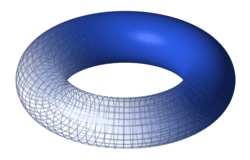# Algebraic topology facts for kids

Kids Encyclopedia FactsA torus, one of the most frequently studied objects in algebraic topology

Algebraic topology is a branch of mathematics that uses tools from abstract algebra to study topological spaces. Algebraic topology can be used in a number of other fields such as physics, branches of geometry and number theory.

Algebraic topology can be used to count "holes" in a shape: for example, a wedding ring and a hollow pipe both have one hole, but a figure-8 has two. This can also be done in different dimensions; a hollow sphere has a kind of "2-dimensional" hole.Algebraic topology Facts for Kids. Kiddle Encyclopedia.